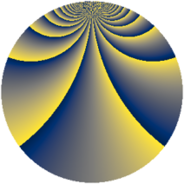# Properties

 Label 2736.2.eqLevel $2736$ Weight $2$ Character orbit 2736.eq Rep. character $\chi_{2736}(1171,\cdot)$ Character field $\Q(\zeta_{12})$ Dimension $792$ Sturm bound $960$

# Related objects

## Defining parameters

 Level: $$N$$ $$=$$ $$2736 = 2^{4} \cdot 3^{2} \cdot 19$$ Weight: $$k$$ $$=$$ $$2$$ Character orbit: $$[\chi]$$ $$=$$ 2736.eq (of order $$12$$ and degree $$4$$) Character conductor: $$\operatorname{cond}(\chi)$$ $$=$$ $$304$$ Character field: $$\Q(\zeta_{12})$$ Sturm bound: $$960$$

## Dimensions

The following table gives the dimensions of various subspaces of $$M_{2}(2736, [\chi])$$.

Total New Old
Modular forms 1952 808 1144
Cusp forms 1888 792 1096
Eisenstein series 64 16 48

## Trace form

 $$792q + 6q^{2} - 2q^{4} + 2q^{5} - 16q^{7} + O(q^{10})$$ $$792q + 6q^{2} - 2q^{4} + 2q^{5} - 16q^{7} + 8q^{11} - 6q^{13} + 18q^{14} - 6q^{16} + 4q^{17} + 4q^{19} - 20q^{20} - 6q^{22} + 4q^{23} - 4q^{26} - 30q^{28} + 6q^{29} - 24q^{32} + 24q^{34} - 12q^{35} - 30q^{38} + 24q^{40} - 2q^{43} + 52q^{44} + 728q^{49} - 54q^{52} + 6q^{53} - 4q^{55} - 36q^{58} + 6q^{59} + 30q^{61} + 62q^{62} - 44q^{64} - 6q^{67} + 8q^{68} + 24q^{70} + 12q^{71} + 50q^{74} + 30q^{76} + 64q^{77} - 14q^{80} + 66q^{82} - 32q^{83} + 34q^{85} + 6q^{86} - 48q^{91} - 26q^{92} - 12q^{97} + 102q^{98} + O(q^{100})$$

## Decomposition of $$S_{2}^{\mathrm{new}}(2736, [\chi])$$ into newform subspaces

The newforms in this space have not yet been added to the LMFDB.

## Decomposition of $$S_{2}^{\mathrm{old}}(2736, [\chi])$$ into lower level spaces

$$S_{2}^{\mathrm{old}}(2736, [\chi]) \cong$$ $$S_{2}^{\mathrm{new}}(304, [\chi])$$$$^{\oplus 3}$$$$\oplus$$$$S_{2}^{\mathrm{new}}(912, [\chi])$$$$^{\oplus 2}$$